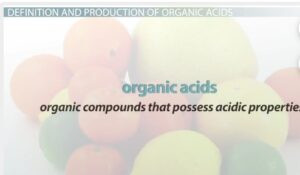You dont have javascript enabled! Please enable it!

# Acid Base And Salt – Bases And Alkalies

ACID BASES AND SALT —BASES AND ALKALISA base is a substance that dissolves in water to produce hydroxide ion OH. This is Arrhenius
definition of base and is called Arrhenius base. Bases are metal oxides and hydroxides.
Examples are:
Base Formula Solubility in water
Sodium oxide Na2O soluble
Calcium oxide CaO Slightly soluble
Potassium oxide K2O Soluble
Sodium hydroxide NaOH Soluble
Potassium hydroxide KOH Soluble
Calcium hydroxide Ca(OH)2 Slightly soluble
Zinc hydroxide Zn(OH)2 Insoluble
ALKALIS: an alkali is a base which is soluble in water (i.e. a soluble base) example are NAOH
and KOH.
NAOH (aq)→ Na ++OH – (aq)

WEAK BASE is a base that ionizes partially in water to produce hydroxide ions. Examples are
ammonia solution (NH4OH) and Ca(OH)2.

Physical Properties of bases Alkalis
1.Concentrated strong base are corrosive.
2.Alkalis/base has a bitter taste
3.They are soapy or slippery to touch.
4.Bases/alkalis turn red litmus paper blue.

#### Chemical Properties of Bases/Alkalis

1.All bases react with acids to produce sal stand water only. This term is called
neutralization reaction
2.Bases react  with ammonium  salt to liberate ammonia gas

NaOH(aq)+NH4Cl(aq)→NaCl(aq)
+H2O(l)+NH3(g)
3.bases react with acid anhydrides to form salt and water.

MEASUREMENT OF ACIDITY AND ALKALINITY (pH)
The pH of a solution is defined as a measure of degree of acidity or alkalinity of a solution.
pH of a solution can also be defined as a negative logarithm to base 10 of hydrogen ion

The concentration of a solution.
pH=-log10[H+]
Instruments Used In Measuring pH
 pH meter
 universal indicator
The pH  Meter: the pH meter is a scale that ranges from numbers 0 to 14
0 1 2 3 4 5 6     7     8 9 10 11 12 13 14
{increasing acidity} from 0 to 6 {neutral} 7 {increasing alkalinity} from 8 to 14
A solution with pH value of 7 is neutral (i.e.not acidic nor alkaline). A solution with pH value of
less than 7 is acidic while pH with values greater than 7 is alkaline.
pH+POH→ 14
[H
+] [OH
-]=10
-14
Calculations involving pH of solution
1.calculate the pH of 0.025 mol/dm3 trioxonitrate (v) solution HNO3
Solution
pH=-log10 [H
+]
=-log10 [2.5×10-2]
=-(-2+0.4)
pH=1.6
2.calculate the pH of 0.1M HNO3 (aq)
Solution
pH=-log10[H
+]
=-log10[0.1]
=-log10[1.0×10
-1]
=-1×-1=1
pH=1.
3. Calculate the pH of a 0.001m acid.
Solution
pH=-log10[H+]
pH=-log10[0.001]
=-log10[1.0×10
-3]
=-(-3)=3
pH=3.
INDICATORS
An acid-base indicator is either a weak organic acid or weak base which gives different colour in acid or alkaline solution. It can also be defined as an organic weak acid or weak base which produces different colours depending on pH of the solution.
Indicators pH range ColourinAcid ColourinBase
Litmus paper 5.0-8.0 Pink/red Blue
Methyl orange 2.9-4.6 Red/Pink Yellow